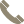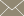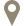Home » Faculty Profiles » Faculty

### San Miguel, Miguel

Lecturer

#### CONTACT INFO(956) 326-2599msanmiguel@tamiu.eduLBV-123

#### COURSES TAUGHT

• Business Math I (MATH 1324)
• Business Math II (MATH 1325)
• Calculus II (MATH 2414)
• Calculus III (MATH 2415)
• College Algebra (MATH 1314)
• Eng Mech Statics & Dynamic Lab (ENGR 2103)
• Introduction to Linear Algebra (MATH 3310)
• Ordinary Diff Equations (MATH 3330)
• Ordinary Differential Equation (MATH 3330)
• Plane Trigonometry (MATH 1316)
• Principles of Elec Engr Lab (ENGR 2105)